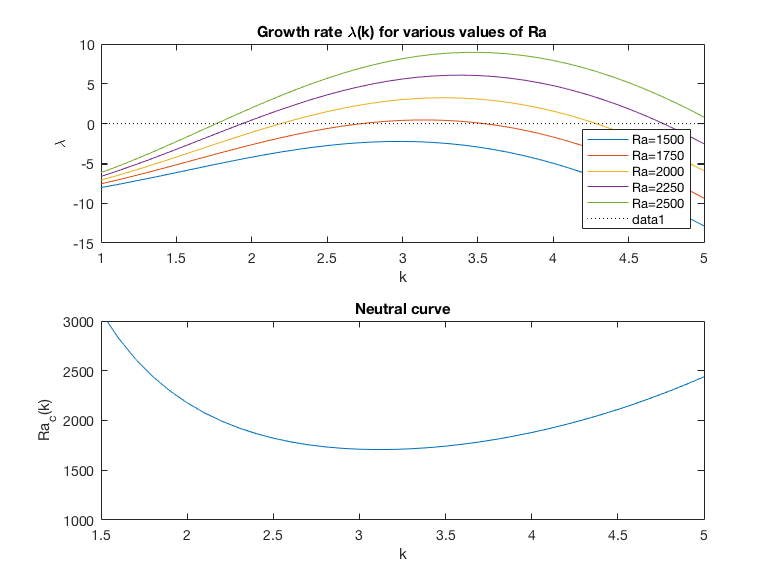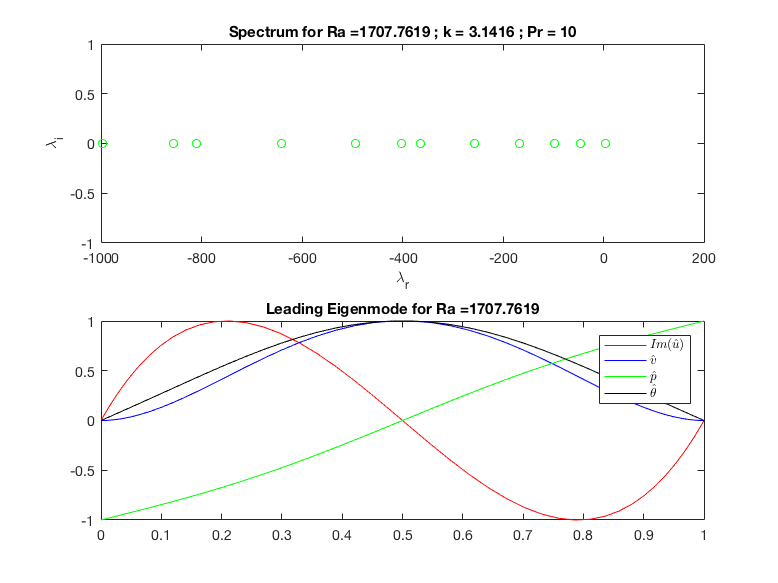# Rayleigh-Benard instability

This is the instability of a fluid layer heated from below. The fluid is sandwiched between two horizontal plates, and the bottom plate is hot and the top plate is cold. Since the hot fluid is lighter, it wants to raise and the cold fluid want to fall. This is hindered by two diffusive effect: the fluid viscosity slows down the motion, and the thermal diffusivity will smear out the temperature.

The important parameters are the fluid viscosity, the distance betwen the two plates, the temperature difference between the two plates, the fluid density, the gravity, the thermal diffusivity and the thermal dilatation \alpha (how much the fluid becomes lighter when it is heated).

This program is adapted from /sandbox/easystab/rayleigh_benard.m, which solved the problem in the case of slip conditions (“Free-Free boundaries”) and validated the approach by comparing with analytical results which exist in this case.

The present program considers the more physical case of no-slip conditions at the walls (“Rigid-Rigid boundaries”). In this case no simple analytical solutions exist, but numerical solutions are given in many books (Drazin & Reid, Chandrasekar, etc…)

function [] = RayleighBenard()

global y dy dyy w Z I N

% parameters
N=50; % number of gridpoints

% differentiation matrices
[dy,dyy,w,y] = dif1D('cheb',0,1,N);
Z=zeros(N,N); I=eye(N);


## Equations

### Starting equations

We start with the Navier-Stokes equations for the fluid, coupled to the heat equation for the temperature and the state equation for an incompresssible, but dilatable fluid. \displaystyle \begin{array}{l} \rho(u_t+uu_x+vu_y)=-p_x+\mu\Delta u\\ \rho(v_t+uv_x+vv_y)=-p_y+\mu\Delta v-\rho g\\ (\rho_t+u \rho_x + v \rho_y) = - \rho (u_x+v_y) \\ T_t+u T_x+v T_y= \kappa \Delta T\\ \rho = \rho_0 \left[ 1 - \alpha (T-T_0) \right] \end{array}

We see that there is the volume force in the v equation, dependent on the density, this will be the term through which the dilatation will affect the flow (the “buoyancy” or “floattability” term, telling how much the fluid “floats” when it is heated).

### Base Flow

The “base flow” solution is the solution of the energy equation in the purely conductive regime :

\displaystyle \overline{T}(y) = T_0 + \frac{(T_1-T_0) y}{H}

The associated density field and pressure field (hydrostatic) are given by :

\displaystyle \overline{\rho}(y) = \rho_0 + \frac{\alpha (T_0-T_1) y}{H}

\displaystyle \overline{P}(y) = P_0 - \int \overline{\rho}(y) g dy

Here \delta T = T_0 - T_1 is the difference of temperature between the lower and upper plates, and assumed positive, and H is the height of the cell.

### Small-perturbation hypothesis and simplifications

We assume that the flow is a small-amplitude deviation to the “base state” previously described :

\displaystyle \left[ \begin{array}{c} u \\ v \\ T \\ \rho \\ p \end{array} \right] = \left[ \begin{array}{c} 0 \\ 0 \\ \overline{T}(y) \\ \overline{\rho}(y) \\\overline{P}(y) \end{array} \right] + \left[ \begin{array}{c} u(x,y,t) \\ v(x,y,t) \\ \theta(x,y,t) \\ \rho'(x,y,t) \\ p'(x,y,t) \\ \end{array} \right]

Under the hypothesis of small perturbations we can do the following simplifications :

• (i) The buoyancy and vertical pressure gradient terms lead to the Boussinesq approximation :

\displaystyle - p_y - \rho g = - p'_y + \rho_0 g \alpha \theta

• (ii) In the NS equations the nonlinear advection terms can be dropped, and \rho can be replaced by \rho_0, except in the buoyancy term

• (iii) The mass equation can be simplified to div( u ) = 0.

• (iv) In the energy equation, the convective derivative is approximated a \displaystyle \frac{d T}{dt} = \theta_t+ v \overline{T}_y

### Resulting set of linearized equations

With these simplifications we end up with the following system of equations :

\displaystyle \begin{array}{l} \rho_0 u_t=-p'_x + \mu\Delta u\\ \rho_0 v_t=-p'_y + \mu\Delta v + \rho_0 \alpha g \theta \\ 0 = u_x+v_y\\ \theta_t = \frac{(T_0-T_1)}{H} v + \kappa \Delta \theta \end{array}

## Numerical resolution for the case of a horizontal cell

We put the equations in nondimensional form by introducing the following dimensionless parameters :

\displaystyle Ra = \frac{g \alpha H^3 (\delta T) }{\nu \kappa} \displaystyle Pr = \frac{\nu}{\kappa}

We thus have the system of four equations

\displaystyle \begin{array}{l} u_t=-p_x + Pr \Delta u,\\ v_t=-p_y + Pr \Delta v + Pr \theta,\\ u_x+v_y=0\\ \theta_t = Ra \, v + \Delta \theta \end{array}

The resolution of this set of equations is done in the function RB.m at the bottom of this program.

## Validation of the code

We first validate the code by looking for the instability threshold.

According to the litterature, the neutral conditions are :

Ra = 1708;
k = 3.117;


These value correspond to a neutral mode whatever the Prandtl number. Here we take :

Pr = 10.


We compute the leading eigenvalue using the function FK defined at the bottom of this program:

lambdamax = RB(k,Ra,Pr)


This value is close to zero. If we want an even better evaluation of the threshold we can use fzero to find the value of Ra where the eigenvalue is exactly zero:

Rac = fzero(@(Ra)(RB(k,Ra,Pr)),Ra)


## Parametric study

We have to characterize the variation of the leading \lambda as function of the two parameters k and Ra (we still consider Pr = 10).

First we compute \lambda(k) for several values of Ra and plot the results in figure 2.

for Ra = [1500:250:2500];
ktab = [1:.1:5];
smaxtab = [];
for k = ktab
[s,U] = RB(k,Ra,Pr);
smaxtab = [smaxtab real(s(1))];
end
figure(2);
subplot(2,1,1);
plot(ktab,smaxtab);hold on;
pause(0.1);
end
plot(ktab,0*ktab,'k:');
xlabel('k');
ylabel('\lambda');
title('Growth rate \lambda(k) for various values of Ra');
legend('Ra=1500','Ra=1750','Ra=2000','Ra=2250','Ra=2500');
legend('Location','SouthEast');
pause(0.1);


We now build the marginal stability curve Ra_c(k) corresponding to the location in the [Ra,k]-plane where the leading eigenvalue is exactly zero.

For this we do a loop over k and look for the location where \lambda_{max} is exactly zero, using again fzero as done above. Results are plotted in figure 2.

    Ra = 3000;
Ratab=[];
ktab = 1.5:.1:5;
for k=ktab
Ra = fzero(@(Ra)(RB(k,Ra,Pr)),Ra);
Ratab= [Ratab Ra];
end

figure(2);
subplot(2,1,2);
plot(ktab,Ratab);
xlabel('k');ylabel('Ra_c(k)');
ylim([1000,3000]);
title('Neutral curve');

set(gcf,'paperpositionmode','auto');
print('-dpng','-r100','RB_neutralcurve.png');The figure

## Spectrum, and structure of the leading eigenmode.

We now take a value of Ra above the threshold and solve the eigenvalue problem for these parameters :

Ra = 2000;
k = pi ;% this means that the wavelenghth is twice the plate spacing)

[s,U] = RB(k,Ra,Pr);


We plot the spectrum and the structure of the leading eigenmode in figure 1

figure(1);
subplot(2,1,1);
plot(real(s),imag(s),'go');
xlabel('\lambda_r');
ylabel('\lambda_i');
title(['Spectrum for Ra =',num2str(Ra),' ; k = ',num2str(k),' ; Pr = ',num2str(Pr)]),

Mode = U(:,1);
subplot(2,1,2);
ModeU = imag(Mode(1:N)); ModeV = real(Mode(N+1:2*N)); ModeP = real(Mode(2*N+1:3*N));ModeT = real(Mode(3*N+1:4*N));
plot(y,ModeU/max(abs(ModeU)),'r',y,ModeV/max(abs(ModeV)),'b',y,ModeP/max(abs(ModeP)),'g',y,ModeT/max(abs(ModeT)),'k');
legend({'$Im(\hat{u})$','$\hat{v}$','$\hat{p}$','$\hat{\theta}$'},'Interpreter','Latex');

set(gcf,'paperpositionmode','auto');
print('-dpng','-r100','RB_spectrumandmode.png');

endThe figure

# FUNCTION RB

Here is the definition of the function RB which performs the eigenvalue
computation. Note that this function is designed so that it can be used
un two ways :

- [s,U] = RB(k,Ra,P) will return a vector s containing the 10 leading
eigenvalues and an array U containing (in column) the corresponding
eigenvectors.

- lambdamax = RB(k,Ra,P) will return only one value corresponding to
the leading eigenvalue. This is useful to use this function with fzero
function [s,U] = RB(k,Ra,Pr)
global y dy dyy w Z I N

% renaming the differentiation matrices
dx=1i*k*I; dxx=-k^2*I;
Delta=dxx+dyy;


## System matrices

We can rewrite the system of equations given in the section #equations in a matrix form \displaystyle Eq_t=Aq with the matrices \displaystyle q=\left(\begin{array}{c} u \\ v\\ p\\ \theta \end{array}\right) , \quad A=\left(\begin{array}{cccc} Pr \Delta&0&-\partial_x&0\\ 0&Pr \Delta&-\partial_y& Pr \\ \partial_x&\partial_y&0&0\\ 0& Ra &0&\Delta \end{array}\right) , \quad E=\left(\begin{array}{cccc}1&0&0&0\\0&1&0&0\\0&0&0&0\\0&0&0&1\end{array}\right)

% system matrices
A=[Pr*Delta, Z, -dx, Z; ...
Z, Pr*Delta, -dy, Pr*I;  ...
dx, dy, Z, Z;  ...
Z, Ra*I, Z, Delta];
E=blkdiag(I,I,Z,I);


## Boundary conditions

The natural conditions are that u and v are zero at the walls, and the temperature perturbation \theta is also zero at the walls (the temperature is imposed at the wall, without perturbations).

\displaystyle \begin{array}{l} u|_0=0\\ u|_L=0\\ v|_0=0\\ v|_L=0\\ \theta|_0=0\\ \theta|_L=0\\ \end{array} thus the boundary conditions are expressed Cq=0, with the constraint matrix

\displaystyle C=\left(\begin{array}{cccc} I|_0&0&0&0\\ I|_L&0&0&0\\ 0&I|_L&0&0\\ 0&I|_0&0&0\\ 0&0&0&I|_L\\ 0&0&0&I|_0\\ \end{array}\right)

% boundary conditions
II=eye(4*N);
u0=1; uL=N; v0=N+1; vL=2*N; T0=3*N+1; TL=4*N;
loc=[u0,uL,v0,vL,T0,TL];
C(loc,:)=II(loc,:);
A(loc,:)=C(loc,:);
E(loc,:)=0;


## Computing eigenmodes

For the linear system Eq_t=Aq we have imposed the boundary conditions in a way that E is not invertible, so we solve a generalized eigenvalue problem which will have infinite eigenvalues corresponding to the boundary conditions constraints. We remove them form the result and we plot only the five eigenvalues that have largest growth rate. These modes will be relevant for the stability analysis. here the wave speed of the mode is zeor, these are stationnary mode, they have no propagation in the x direction, so we plot only the growth rate.

% computing eigenmodes
[U,S]=eig(A,E);
s=diag(S);  [t,o]=sort(-real(s)); s=s(o); U=U(:,o);
rem=abs(s)>1000; s(rem)=[]; U(:,rem)=[];

if(nargout==1)
s=real(s(1));
% this is a trick to allow to use the function as s=RT(k,Ra,Pr).
% This allows to pass the function as a handle for fzero.
end

end


# Exercices/Contributions

• Please superpose the neutral curve onto the results of Drazin & Reid (Fig. 2.2, page 53)
• Drazin & Reid (page 51) found that a second mode becomes unstable above a critical Rayleigh number of 17610 for k=5.365. Please check these results with the present code.
• Please show that the flow is always stable when the hot plate is at the top
• Please draw the velocity field and the temperature field corresponding to the five most unstable modes. (this is done for the unphysical case with “slip” conditions in this program : /sandbox/easystab/stab2014/rayleigh_benard_profil_temperature.m )

• Please write a code that does the advection of tracer particles by the velocity field of the first eigenmode in a stable case and in an unstable case
• Extension considering surface tension and a free surface, also called Rayleigh Benard Marangoni convection